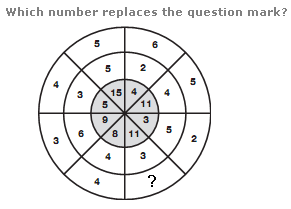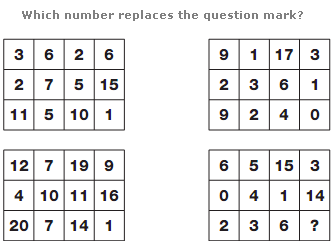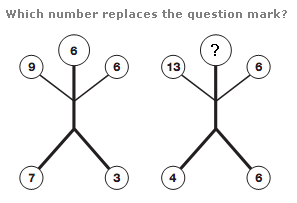# Puzzles - Number puzzles

### Exercise :: Number puzzlesAnswer : 7 Explanation : In each segment of the circle, multiply the outer 2 numbers together and subtract the sum of the outer 2 numbers in the opposite segment to give the value in the inner grey segment.Answer : 1 Explanation : Values in the lower left table equal the sums of the values in corresponding positions of the top two tables, and values in the lower right table equal the difference in values in corresponding positions of the top two tables.Answer : 5 Explanation : In each diagram, the sum of the three upper numbers equals the product of the two lower numbers.